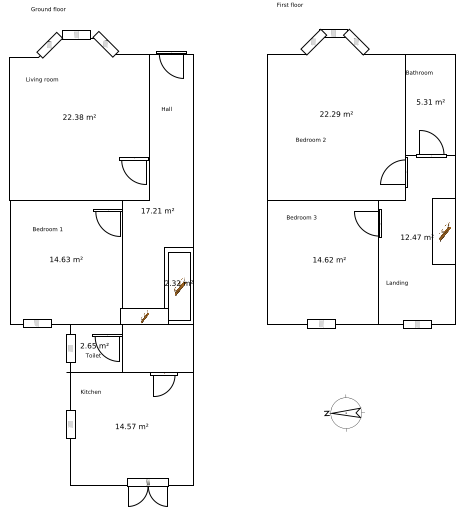# Declarative modelling

Simile is billed as a “visual modelling environment”, meaning that models are developed diagrammatically (as opposed to writing lines of text, as in a programming language or a simulation language). However, a more fundamental feature of Simile is that it is a declarative modelling environment. In a sense, Simile’s visual modelling interface is just one way of building a Simile model: we could envisage other interfaces (such as a text editor) that would still produce a Simile model, but would not be visual.

What do we mean by “declarative modelling”? First, we note that the term contrasts with a procedural approach, which is what we do when we implement a model in a conventional (procedural) programming language, such as Fortran or C. In that case the lines in the program are in fact instructions to the computer: calculate this value, loop over the array elements, print the value for this variable. Some of the lines might look exactly like declaratively-expressed equations, but they are in fact assignment statements. Thus, without the use of strictly-observed (and arbitrary) conventions, we cannot really distinguish between the assignment statements in the program that constitute the model equations, and all the other assignments we might have in the program. Effectively, we can do only two things with the program: run it (to calculate the values for the model variables); or show it to a human who understands the programming language. And running it is the only thing that a computer can do for us.

In declarative modelling, we represent a model not as a series of assignment and control statements, but as a set of facts that are true about the model. The order in which we present the facts is (unlike a procedural program) irrelevant. The full set of facts defining a model actually constitute a specification for the model: given these facts, and knowledge about what the symbols mean, someone else can construct a working version of the models.

A good example is in architectural design. We would never dream of specifying adesign for a building in a computer program: rather, we would express it as a set of facts, defining the walls, doors etc, and their size, location and materials. Given this information, we could draw a plan for the building. We also have all the information we need to do calculations about the building: how big it is; how quickly it would heat up and cool down. The program that does the calculations is indeed procedural; but the design of a particular building is held as a set of facts.

And so it can be with models. A model is a certainly a design, expressed in terms of the constituent parts of the model and the linkages between them. It can certainly be expressed declaratively: look at he “Model description” part of any modelling paper. The declarative statement of model structure is sufficient to enable someone else to make a runnable version of the model, provided that it is complete, unambiguous, and that the meaning of the terms used is understood.